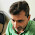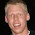## Sunday, March 9, 2014

### Custom OpenCL functions in C++ with Boost.Compute

Due to OpenCL's C99-based programming and compilation model, defining and using custom functions from C++ on the GPU can be challenging. However, Boost.Compute provides a few utilities to simplify function creation and execution from C++ (without ever having to touch a raw source code string!).

The BOOST_COMPUTE_FUNCTION() macro creates function objects in C++ which can then be executed on the GPU by OpenCL with the Boost.Compute algorithms (e.g. transform(), sort()).

As arguments, the macro takes the function's return type, name, argument list, and source. The first three arguments (return type, name, and argument list) are all C++ types/expressions. The source argument contains the body of the function which will be inserted into an OpenCL program when executed with one of the Boost.Compute algorithms.

The return type, name, and argument list are used by Boost.Compute to automatically generate the OpenCL function declaration as well as to instantiate the C++ function<> object with the correct signature. This ensures type-safety in C++ (e.g. calling the function with the wrong number of arguments will result in a C++ compile-time error).

The following example shows how to create a comparison function which can be passed to the sort() algorithm in order to sort a list of vectors by their length.

The BOOST_COMPUTE_CLOSURE() is similar to the function macro but additionally allows for a set of C++ variables to be "captured" and then used in the OpenCL function source. This is similar to passing variables to C++11 lambda functions with the capture list. For now, only value types (e.g. float, int4) can be captured. In the future I plan on extending this to allow memory-object types (e.g. buffer, image2d) as well.

The following example shows how to create a function which determines if a 2D cartesian point is contained in a circle with its radius and center given in C++.

As you can see, the C++ center and radius variables have been captured by the closure function and made available for use in the OpenCL source code. Under the hood this is accomplished by invisibly passing the captured values to OpenCL when the function is invoked.

In addition to these macros, Boost.Compute also contains a lambda-expression framework which allows for one-line C++ expressions to be converted to OpenCL source-code and executed on the GPU. This is similar to the Boost.Lambda library and based on the Boost.Proto library.

The previous example showing how to sort vectors by their length could also be written using a lambda-expression as follows:

Together these macros and the lambda-expression framework provide a powerful way to create OpenCL functions interspersed with C++ code (and, notably, doesn't require a special compiler or any non-standard compiler extensions!).

Edit: As of this commit on 4/20/2014 the BOOST_COMPUTE_FUNCTION() macro now uses a list of arguments including their type and name. The old, auto-generated names (e.g. _1, _2) are no longer used. The new version allows for clearer code with more descriptive variable names. The examples above have been updated to reflect the new API.

1.> The function variables are given the placeholder names _1, _2, ... _n (in the future I plan on allowing custom parameter names to be specified as well).

I'd like to have that in VexCL as well. Named parameters could make longer function bodies much more readable. The drawback is that I can not come up with a concise syntax for giving parameter names (which would not make defining shorter functions a hassle). Do you have any ideas here?

Another problem (that I would like to see an elegant solution for) with user-defined functions: it seems impossible to call a user-defined function from another function's body (because the body is just a string, opaque for the host compiler) without introducing more hoops for the user.

1.Both are issues I've though about (and both should be solvable :-) ).

My idea for named parameters is to allow the function macro to accept a list of (type, name) pairs for the function arguments argument. So something like BOOST_COMPUTE_FUNCTION(bool, foo, (int, int), ...) would get the default '_1' and '_2' argument names while BOOST_COMPUTE_FUNCTION(bool, bar, ((int, x), (int, y)), ...) would get 'x' and 'y' as the argument names. This should be doable using a bit of Boost.PP magic.

Supporting functions that call other functions is a bit trickier. I don't think it's possible to support automatically (though maybe it is with C++11 user-defined-literals, I'll have to investigate more). My general plan is to add something like a 'depends_on(Function)' method to the function<> class which is called by the user to indicate that one function depends on another (though possible this could be wrapped up with some meta-programming and resolved at compile-time). Then Boost.Compute would ensure that all of a functions dependencies are inserted before the function itself is used. This will take a bit more time to plan out and implement. However, supporting functions that depend on other functions will allow for more general/generic OpenCL/C++ function libraries to be created and provided to users. This is definitely on my TODO list.

Any other ideas you've had?

2.I like the idea with preprocessor lists; I'll probably have to look more at Boost.PP possibilities.

Regarding the 'depends_on()' solution to the second problem: this could work for Boost.Compute, because (a) your hands are not tied with requirement that kernels should be distinguishable at compile time, and (b) you don't invent your own name for the function (user provides the name, hence he knows what to call).

Second issue is the bigger one for me, because I don't ask users for the function name.

I did something similar to what you propose (but more explicit) when I implemented sort/reduce primitives from http://nvlabs.github.io/moderngpu. The code is heavily based on templated device functions, and for each of those I had two methods: one for inserting the function call into kernel code, and the other to insert the definition of the function into the kernel preamble. The name of the actual function had to be generated because single kernel may use several instantiations of a single templated function (see e.g. here). Then, at the kernel generation site I explicitly state which functions will I need.

2.Hi,
I am using boost compute and i have the following question.
My goal is to apply a transform function (T1) on a vector (V1) and Put the values in a vector (V2). As far as my understanding goes if i use boost::compute::transform it will apply on each element T1 function on each element of V1 and puts the corresponding value in V2,
Now i want my T1 function not only take an element of the vector V1 as input but also another input let us say L1 which is instance of struct
with value set accordingly . And this input remains same for T1 even though the its another input moves over each element in the vector . How to do this efficently in boost compute ?
One solution is to use another vector which has L1 values repeated and then apply a binary operator, but that sounds inefficent. Is there any pther way to achieve this.

thanks

3.L1 should be part of the closure as it is center and radius on the example.

4.Is it possible to write the BOOST_COMPUTE_FUNCTION to sort using just a selected element within the float4_ vectors? At present I am getting an error that says there is no .x member within the float4_ type (I'm used to OpenCL float4 which does allow this)

e.g.
BOOST_COMPUTE_FUNCTION(bool, compare_x, (float4_ a, float4_ b),
{
return a.x < b.x;
});

1.Yes, this is possible. For an example, see the "sort_int2" test-case in test_stable_sort.cpp (https://github.com/boostorg/compute/blob/master/test/test_stable_sort.cpp#L41).

5.Bingo, I missed that as I only read through all the examples and not the test file. Thanks!

I have a working sketch now but if I go over 4096 elements in the vector I get an openCl 'out of resources error' & crash. Am guessing since that is 64sq this might be a workgroup issue; so do large arrays have to be divided up? 64 seems a low max workgroup, but I am probably missing something else

6.This comment has been removed by the author.

7.Hi,
I try to use BOOST_COMPUTE_CLOSURE with min_element(), like this:

struct f3
{
float x, y, z;
};
BOOST_COMPUTE_ADAPT_STRUCT(f3, f3, (x, y, z));
...
float x0 = ...
float y0 = ...
BOOST_COMPUTE_CLOSURE(bool, min_dst, (f3 a, f3 b), (x0, y0),
{
float ax = a.x - x0;
float ay = a.y - y0;
float bx = b.x - x0;
float by = b.y - y0;
return ax * ax + ay * ay < bx * bx + by * by;
});
auto mini = boost::compute::min_element(first, last, min_dst, queue);

I get opencl_error "Build Program Failure".
What do I do wrong?

1.Sorry for the double commit.

I think I've found the problem:

Index: find_extrema_with_reduce.hpp
===================================================================
--- find_extrema_with_reduce.hpp (revision 37940)
+++ find_extrema_with_reduce.hpp (working copy)
@@ -128,7 +128,7 @@
// Next element
k.decl("next") << " = " << input[k.var("idx")] << ";\n" <<
"#ifdef BOOST_COMPUTE_USE_INPUT_IDX\n" <<
- k.decl("next_idx") << " = " << input_idx[k.var("idx")] << ";\n" <<
+ k.decl("next_idx") << " = " << input_idx[k.var("idx")] << ";\n" <<
"#endif\n" <<

// Comparison between currently best element (acc) and next element

8.This comment has been removed by the author.

9.Hmmm, I'n not a very professional comment writer. The main point is to use uint_ instead of input_type as the template parameter.

- k.decl _ input_type _ ("next_idx") << " = " << input_idx[k.var("idx")] << ";\n" <<
+ k.decl _ uint_ _("next_idx") << " = " << input_idx[k.var("idx")] << ";\n" <<

1.Thanks for the report! Would you mind opening an issue for this on GitHub: https://github.com/boostorg/compute/issues.

2.It has already been corrected on https://github.com/boostorg/compute

10.Have you ever thought about adding a little bit more than just your articles? I mean, what you say is important and all. duo queue boost

11.This is a decent post. This post give really quality data. I'm certainly going to investigate it. Truly exceptionally valuable tips are shown here. much obliged to you to this kind of extent. Maintain the acts of kindness.elo boost